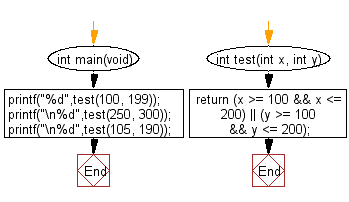﻿ C Program: Check if two integers in the range 100..200# C Exercises: Check two given integers whether either of them is in the range 100..200 inclusive

## C-programming basic algorithm: Exercise-7 with Solution

Write a C program to check two given integers whether either of them is in the range 100..200 inclusive.

C Code:

``````#include <stdio.h>
#include <stdlib.h>
int main(void){
printf("%d",test(100, 199));
printf("\n%d",test(250, 300));
printf("\n%d",test(105, 190));
}
int test(int x, int y)
{
return (x >= 100 && x <= 200) || (y >= 100 && y <= 200);
}
``````

Sample Output:

```1
0
1
```

Pictorial Presentation:Flowchart:C Programming Code Editor:

What is the difficulty level of this exercise?

Test your Programming skills with w3resource's quiz.

﻿

## C Programming: Tips of the Day

Why doesn't a+++++b work?

printf("%d",a+++++b); is interpreted as (a++)++ + b according to the Maximal Munch Rule!.

++ (postfix) doesn't evaluate to an lvalue but it requires its operand to be an lvalue.

! 6.4/4 says the next preprocessing token is the longest sequence of characters that could constitute a preprocessing token"

Ref : https://bit.ly/3fdldUT